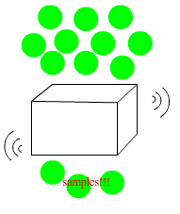## FANDOM

116 Pages

Random sampling, which is also called simple random sampling, is the most basicand straightforward sampling method used by the sociologists. In a simple random sample, each member of the selected population has a equal chance of being selected as part of the sample, and each subset of k individuals has the same probability of being chosen for the sample as any other subset of k individuals. Therefore, it removes bias from the procedure and should gives out a representative sample. It is one of the most popular sampling methods as it is easy to operate.

## ​Application

How to appropriately use the random sampling method? Follow the next few steps:

1.Prepare a list of all population involved. Number each of the member from 1 to N (N is the population size).

2.Choose n items from a list of N. This can be done using a computer software, a random number table or other methods that can generate random numbers. There are two popular approaches that are aimed to minimize the relevance of bias in the process of random sampling selection: method of lottery and the use of random numbers.

In method of lottery you will have to number each member of population with a consequent manner, writing numbers in separate pieces of paper. These pieces of papers are to be folded and mixed into a box. Lastly, samples are to be taken randomly from the box by choosing folded pieces of papers in a random manner.

The use of random numbers, an alternative method also involves numbering of population members from 1 to N. Then, the sample size of has to be determined by selecting numbers randomly. The use of random number table similar to one below can help greatly with the application of this sampling technique.

3.Look back to the population list, and find out the corresponding member name of the selected number. Get them involved in your research!

1. If applied appropriately, simple random sampling is associated with the minimum amount of sampling bias compared to other sampling methods. Research findings resulting from the application of simple random sampling can be generalized due to representativeness of this sampling technique and a little relevance of bias.
2. Given the large sample frame is available, the ease of forming the sample group i.e. selecting samples is one of the main advantages of simple random sampling. Unlike more complicated sampling methods such as stratified random sampling and probability sampling, no need exists to divide the population into subpopulations or take any other additional steps before selecting members of the population at random.